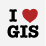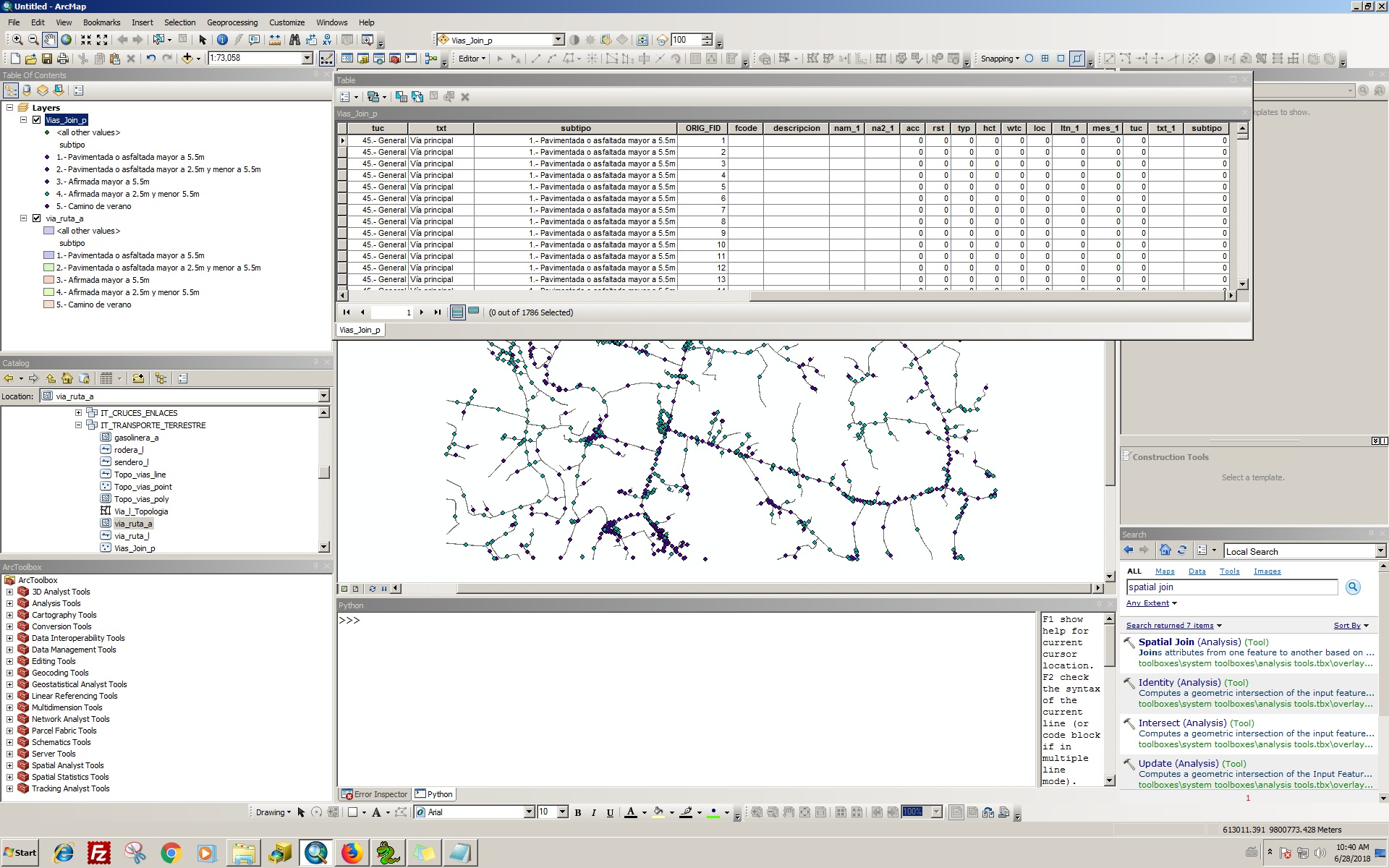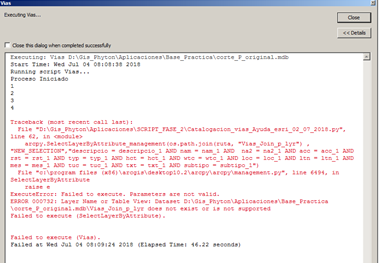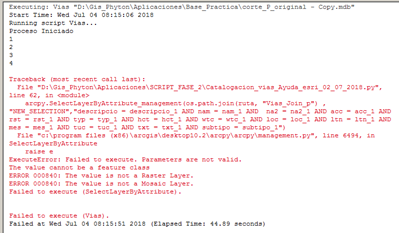# Problemas Spatial Join con script en Python

1239
11
06-28-2018 08:49 AMNew Contributor II

Buen dia amigos,

```Gracias a aceptarme en la comunidad. Tengo un problema. Estoy intentando hacer un script, donde el objetivo es realizar es una unión espacial en mi caso. entre target = Vias_Join_p (punto de camino) y Join = via_ruta_a (polígono de camino)  Esta es la línea, se ejecuta bien en la ventana de comandos en ArcMap, pero cuando intento ejecutar Pythonwin o ejecutar un script en la caja de herramientas, no aparece la unión, solo aparece campos de via_ruta_a con valor 0 en la tabla de Vias_Join_p.  arcpy.SpatialJoin_analysis ("vias_l_p", "via_ruta_a", os.path.join (ruta, "IT_TRANSPORTE_TERRESTRE", "Vias_Join_p"), "JOIN_ONE_TO_ONE", "KEEP_ALL", "", "INTERSECT")  Las dos coberturas tienen dominios en sus campo, no se si esto sea un inconveniente, adjunto una imagen con la tabla de resultados.
gracias por las respuestas!```
Tags (3)
1 Solution

Accepted SolutionsbyMVP Regular Contributor

The first few lines of the error message indicate the error occurred at (or just before) line 60 in your code, and it gives the text of the line in question.

``````File "D:\Gis_Phyton\Aplicaciones\SCRIPT_FASE_2\Catalogacion_vias_Ayuda_esri_04_07_2018.py", line 60, in <module>
arcpy.SpatialJoin_analysis(os.path.join(ruta, "vias_l_p"),os.path.join(ruta, "via_ruta_a"),os.path.join(ruta, "Vias_Join_p"),"JOIN_ONE_TO_ONE","KEEP_ALL","","INTERSECT")
‍‍``````

Then the error message indicates a line number in the SpatialJoin module where the error was triggered.   Then the message indicates the specific nature of the problem.

``````ExecuteError: Failed to execute. Parameters are not valid.
ERROR 000732: Target Features: Dataset D:\Gis_Phyton\Aplicaciones\Base_Practica\MEJIA_RUMINAHUI_WGS84_17S_5000.mdb\vias_l_p does not exist or is not supported
‍‍``````

In this case, it is suggesting a couple of things.  First, the Access database (a personal geodatabase) does not contain a feature named "vias_l_p" or the database does not exist.  In this case, check that the workspace, path and feature name are correct.

If the path and feature name is correct, then check to see if the type of join you are trying to accomplish is permitted - that includes making sure the  "vias_l_p" feature is the correct type, points, etc.

11 RepliesbyMVP Regular Contributor

(an approximate translation to English)

Good day friends,

Thank you to accept me in the community. I have a problem. I'm trying to make a script, where the goal is to realize is a spatial union in my case. between target = Vias_Join_p (path point) and Join = via_ruta_a (path polygon) This is the line, it runs well in the command window in ArcMap, but when I try to run Pythonwin or run a script in the toolbox, it does not union appears, only fields of via_ruta_a with value 0 appear in the table of Vias_Join_p.

``arcpy.SpatialJoin_analysis("vias_l_p", "via_route_a", os.path.join (path, "IT_TRANSPORTE_TERRESTRE", "Vias_Join_p"), "JOIN_ONE_TO_ONE", "KEEP_ALL", "", "INTERSECT")‍‍``

The two coverages have domains in the field, I do not know if this is an inconvenience, I attach an image with the results table.New Contributor II
`Thanks for the translation!Now, I hope that someone help me with my problem `byMVP Regular Contributor

In your script, are you using the full path to your target and join features (or an arcpy.env.workspace setting)?  Are you receiving any error messages when using Pythonwin; if so, what are they?  Can you share a bit more of your script so we can see how the target and join features are initialized before the SpatialJoin.  Thanks.New Contributor II

Hi Randy, here is the script

import arcpy
import os

# Definicion del espacio de trabajo
arcpy.overwriteOutput = True
ruta = arcpy.env.workspace = arcpy.GetParameterAsText(0)

datasets = arcpy.ListDatasets(feature_type='feature')
datasets = [''] + datasets if datasets is not None else []
for ds in datasets:
for fc in arcpy.ListFeatureClasses(feature_dataset=ds):
#if fc == "via_ruta_l":

if  ds == "IT_TRANSPORTE_TERRESTRE" and fc == "via_ruta_l":
input_dataset = ds
input_Fclass  = fc
topo_name = "Via_l_Topologia"
cluster_tol = 0.001
topoReglas = ["Must Not Intersect (Line)","Must Not Self-Intersect (Line)","Must Not Intersect Or Touch Interior (Line)"]
validate = "true"

out_topo = arcpy.CreateTopology_management(input_dataset, topo_name, cluster_tol)

print(arcpy.GetMessages())
arcpy.ValidateTopology_management(out_topo)
arcpy.ExportTopologyErrors_management(out_topo, ds, "Topo_vias")

arcpy.MakeFeatureLayer_management("via_ruta_l","via_ruta_l_lyr")
arcpy.MakeFeatureLayer_management("Topo_vias_point","Topo_vias_point_lyr")

arcpy.SelectLayerByLocation_management("via_ruta_l_lyr", "INTERSECT", "Topo_vias_point_lyr")
arcpy.SplitLineAtPoint_management("via_ruta_l_lyr","Topo_vias_point_lyr",os.path.join(ruta,"IT_TRANSPORTE_TERRESTRE","vias_l_c") ,"0.1 Meters")

arcpy.MakeFeatureLayer_management("vias_l_c","vias_l_c_lyr")
arcpy.SelectLayerByLocation_management("via_ruta_l_lyr", "INTERSECT", "Topo_vias_point_lyr","","SWITCH_SELECTION")

arcpy.Append_management("via_ruta_l_lyr" , "vias_l_c_lyr" , "NO_TEST", " "," " )

arcpy.FeatureToPoint_management("vias_l_c_lyr",os.path.join(ruta,"IT_TRANSPORTE_TERRESTRE","vias_l_p"),"INSIDE")

elif fc ==  "via_ruta_a":
arcpy.SpatialJoin_analysis("vias_l_p","via_ruta_a",os.path.join(ruta,"IT_TRANSPORTE_TERRESTRE","Vias_Join_p"),"JOIN_ONE_TO_ONE","KEEP_ALL","","INTERSECT")

# the goal of this script is compare each field, here are another line that didn´t do anything

#arcpy.SelectLayerByAttribute_management("Vias_Join_p_lyr","NEW_SELECTION","descripcio" == "descripcio_1" and "nam" == "nam_1" and  "na2" == "na2_1" and "acc" == "acc_1" and "rst" == "rst_1" and "typ" == "typ_1" and "hct" == "hct_1" and "wtc" == "wtc_1" and "loc" == "loc_1" and "ltn" == "ltn_1" and "mes" == "mes_1" and "tuc" == "tuc_1" and "txt" == "txt_1" and "subtipo" == "subtipo_1")byMVP Regular Contributor

Thanks for posting your code.  I noticed a couple of things.

First, to your original question.  You are indicating that the scrip works well inside ArcMap, but not outside.  I suspect it may be with the workspace path that is being created.  I do not know if "IT_TRANSPORTE_TERRESTRE" is part of what is assigned to the workspace variable "ruta" or not.

``````ruta = arcpy.env.workspace = arcpy.GetParameterAsText(0)

arcpy.SpatialJoin_analysis(
"vias_l_p",
"via_ruta_a",
os.path.join(ruta,"IT_TRANSPORTE_TERRESTRE","Vias_Join_p"),
"JOIN_ONE_TO_ONE",
"KEEP_ALL",
"",
"INTERSECT"
)

# assuming workspace is:  \Some\Path\IT_TRANSPORTE_TERRESTRE
# my suggestion is to try:

arcpy.SpatialJoin_analysis(
os.path.join(ruta, "vias_l_p"),
os.path.join(ruta, "via_ruta_a"),
os.path.join(ruta, "Vias_Join_p"),
....

# or try

arcpy.SpatialJoin_analysis(
"vias_l_p",
"via_ruta_a",
"Vias_Join_p",
....

# assuming workspace is:  \Some\Path\
# (does not include "IT_TRANSPORTE_TERRESTRE"
# my suggestion is to try:

arcpy.SpatialJoin_analysis(
os.path.join(ruta,"IT_TRANSPORTE_TERRESTRE","vias_l_p"),
os.path.join(ruta,"IT_TRANSPORTE_TERRESTRE","via_ruta_a"),
os.path.join(ruta,"IT_TRANSPORTE_TERRESTRE","Vias_Join_p"),
....‍‍‍‍‍‍‍‍‍‍‍‍‍‍‍‍‍‍‍‍‍‍‍‍‍‍‍‍‍‍‍‍‍‍‍‍‍‍‍‍‍‍‍‍‍‍‍‍‍‍‍‍‍‍‍‍‍‍‍‍‍‍‍‍‍‍‍‍‍‍‍‍‍‍‍‍‍‍‍‍‍‍‍‍‍‍‍‍‍‍‍‍‍‍‍‍‍‍‍‍‍‍‍‍‍‍``````

In your code (at the end) you mentioned having a problem with another line.  Here the problem looks like an incorrectly formed where clause.  Often the field names should not be quoted, and in SQL the equality symbol is just a single "=".  If the SQL server you are using requires quoting field names then you will need to escape them.  You should check the documentation for your server.

``````# another line that didn´t do anything

arcpy.SelectLayerByAttribute_management(
"Vias_Join_p_lyr",
"NEW_SELECTION",
"descripcio" == "descripcio_1" and "nam" == "nam_1" and  "na2" == "na2_1" and "acc" == "acc_1" and "rst" == "rst_1" and "typ" == "typ_1" and "hct" == "hct_1" and "wtc" == "wtc_1" and "loc" == "loc_1" and "ltn" == "ltn_1" and "mes" == "mes_1" and "tuc" == "tuc_1" and "txt" == "txt_1" and "subtipo" == "subtipo_1")

# for where_clause try:

"descripcio = descripcio_1 AND nam = nam_1 AND  na2 = na2_1" # continue ....

# if field names need quotes, escape them using \"

"\"descripcio\" = \"descripcio_1\" AND \"nam = nam_1\" AND  \"na2\" = \"na2_1\"" # continue ....‍‍‍‍‍‍‍‍‍‍‍‍‍‍``````

If after you try these suggestions, you are still having problems then be sure to include any error messages in your post.

Hope this helps.New Contributor II

import arcpy
import os

# Definicion del espacio de trabajo
arcpy.overwriteOutput = True
ruta = arcpy.env.workspace = arcpy.GetParameterAsText(0)

datasets = arcpy.ListDatasets(feature_type='feature')
datasets = [''] + datasets if datasets is not None else []
for ds in datasets:
for fc in arcpy.ListFeatureClasses(feature_dataset=ds):
#if fc == "via_ruta_l":
#print fc

if  ds == "IT_TRANSPORTE_TERRESTRE" and fc == "via_ruta_l":
input_dataset = ds
input_Fclass  = fc
topo_name = "Via_l_Topologia"
cluster_tol = 0.001
topoReglas = ["Must Not Intersect (Line)","Must Not Self-Intersect (Line)","Must Not Intersect Or Touch Interior (Line)"]
validate = "true"

#Crear topologia de Altimetría
out_topo = arcpy.CreateTopology_management(input_dataset, topo_name, cluster_tol)

print(arcpy.GetMessages())
arcpy.ValidateTopology_management(out_topo)
arcpy.ExportTopologyErrors_management(out_topo, ds, "Topo_vias")

arcpy.MakeFeatureLayer_management("via_ruta_l","via_ruta_l_lyr")
arcpy.MakeFeatureLayer_management("Topo_vias_point","Topo_vias_point_lyr")

arcpy.SelectLayerByLocation_management("via_ruta_l_lyr", "INTERSECT", "Topo_vias_point_lyr")
arcpy.SplitLineAtPoint_management("via_ruta_l_lyr","Topo_vias_point_lyr",os.path.join(ruta,"IT_TRANSPORTE_TERRESTRE","vias_l_c") ,"0.1 Meters")

arcpy.MakeFeatureLayer_management("vias_l_c","vias_l_c_lyr")
arcpy.SelectLayerByLocation_management("via_ruta_l_lyr", "INTERSECT", "Topo_vias_point_lyr","","SWITCH_SELECTION")

arcpy.Append_management("via_ruta_l_lyr" , "vias_l_c_lyr" , "NO_TEST", " "," " )

arcpy.FeatureToPoint_management("vias_l_c_lyr",os.path.join(ruta,"IT_TRANSPORTE_TERRESTRE","vias_l_p"),"INSIDE")
#arcpy.MakeFeatureLayer_management("vias_l_p","vias_l_p_lyr")

elif fc ==  "via_ruta_a":
arcpy.SpatialJoin_analysis(os.path.join(ruta, "vias_l_p"),os.path.join(ruta, "via_ruta_a"),os.path.join(ruta, "Vias_Join_p"),"JOIN_ONE_TO_ONE","KEEP_ALL","","INTERSECT")

#####New Problem when I put this lines, I have an error, that "Vias_Join_p_lyr" its is not supported ###

arcpy.MakeFeatureLayer_management(os.path.join(ruta,"Vias_Join_p"),(os.path.join(ruta, "Vias_Join_p_lyr")))
arcpy.SelectLayerByAttribute_management(os.path.join(ruta, "Vias_Join_p_lyr") , "NEW_SELECTION","descripcio = descripcio_1 AND nam = nam_1 AND  na2 = na2_1 AND acc = acc_1 AND rst = rst_1 AND typ = typ_1 AND hct = hct_1 AND wtc = wtc_1 AND loc = loc_1 AND ltn = ltn_1 AND mes = mes_1 AND tuc = tuc_1 AND txt = txt_1 AND subtipo = subtipo_1")
arcpy.SelectLayerByAttribute_management("Vias_Join_p_lyr" , "SWITCH_SELECTION","")### When I put this lines I have another error
arcpy.SelectLayerByAttribute_management(os.path.join(ruta, "Vias_Join_p") , "NEW_SELECTION","descripcio = descripcio_1 AND nam = nam_1 AND  na2 = na2_1 AND acc = acc_1 AND rst = rst_1 AND typ = typ_1 AND hct = hct_1 AND wtc = wtc_1 AND loc = loc_1 AND ltn = ltn_1 AND mes = mes_1 AND tuc = tuc_1 AND txt = txt_1 AND subtipo = subtipo_1")

arcpy.SelectLayerByAttribute_management("Vias_Join_p" , "SWITCH_SELECTION","")Please Could you help me? I have tried of some ways, but I dont have a good resultbyMVP Honored Contributor

The output of MakeFeatureLayer is a feature layer, not a file, so don't include the path. Then, the input to SelectLayerByAttribute is a feature layer, so again, no file path.

``````arcpy.MakeFeatureLayer_management(os.path.join(ruta,"Vias_Join_p"), "Vias_Join_p_lyr")
arcpy.SelectLayerByAttribute_management("Vias_Join_p_lyr" , "NEW_SELECTION","descripcio = descripcio_1 AND nam = nam_1 AND  na2 = na2_1 AND acc = acc_1 AND rst = rst_1 AND typ = typ_1 AND hct = hct_1 AND wtc = wtc_1 AND loc = loc_1 AND ltn = ltn_1 AND mes = mes_1 AND tuc = tuc_1 AND txt = txt_1 AND subtipo = subtipo_1")‍‍‍‍``````New Contributor II

I have a question  how arcpy works I reffer about the lines of programation I´ll trying to understand it, because in some GDB the script that I did run very well, but in some cases it doesn´t work, and the error is in a specific line, and dosn´t do previous line of instruction .

## Error here we have an  error that I run the script in another GDB, for me its so rare because it have the same structure, feature data sets, featureclass, I dont know what is the origen of the problem, I was Thinking that could be the version of the GDB  9.3 o 10.2 or another, but Ithe script runs well in two versions, and that it make crazyat the end I ´ll poste the complete script Thanks for you help##

Traceback (most recent call last):
File "D:\Gis_Phyton\Aplicaciones\SCRIPT_FASE_2\Catalogacion_vias_Ayuda_esri_04_07_2018.py", line 60, in <module>
arcpy.SpatialJoin_analysis(os.path.join(ruta, "vias_l_p"),os.path.join(ruta, "via_ruta_a"),os.path.join(ruta, "Vias_Join_p"),"JOIN_ONE_TO_ONE","KEEP_ALL","","INTERSECT")
File "C:\Program Files (x86)\ArcGIS\Desktop10.2\arcpy\arcpy\analysis.py", line 485, in SpatialJoin
raise e
ExecuteError: Failed to execute. Parameters are not valid.
ERROR 000732: Target Features: Dataset D:\Gis_Phyton\Aplicaciones\Base_Practica\MEJIA_RUMINAHUI_WGS84_17S_5000.mdb\vias_l_p does not exist or is not supported
Failed to execute (SpatialJoin).

Failed to execute (Vias).

### Script ###

import arcpy
import os

# Definicion del espacio de trabajo
arcpy.overwriteOutput = True
ruta = arcpy.env.workspace = arcpy.GetParameterAsText(0)

datasets = arcpy.ListDatasets(feature_type='feature')
datasets = [''] + datasets if datasets is not None else []
for ds in datasets:
for fc in arcpy.ListFeatureClasses(feature_dataset=ds):
#if fc == "via_ruta_l":
#print fc

if  ds == "IT_TRANSPORTE_TERRESTRE" and fc == "via_ruta_l":
input_dataset = ds
input_Fclass  = fc
topo_name = "Via_l_Topologia"
cluster_tol = 0.001
topoReglas = ["Must Not Intersect (Line)","Must Not Self-Intersect (Line)","Must Not Intersect Or Touch Interior (Line)"]
validate = "true"

#Crear topologia de Altimetría
out_topo = arcpy.CreateTopology_management(input_dataset, topo_name, cluster_tol)

print(arcpy.GetMessages())
arcpy.ValidateTopology_management(out_topo)
arcpy.ExportTopologyErrors_management(out_topo, ds, "Topo_vias")

arcpy.MakeFeatureLayer_management("via_ruta_l","via_ruta_l_lyr")
arcpy.MakeFeatureLayer_management("Topo_vias_point","Topo_vias_point_lyr")

arcpy.SelectLayerByLocation_management("via_ruta_l_lyr", "INTERSECT", "Topo_vias_point_lyr")

arcpy.FeatureClassToFeatureClass_conversion("via_ruta_l",os.path.join(ruta,"IT_TRANSPORTE_TERRESTRE"), "vias_l_parcial")
arcpy.MakeFeatureLayer_management("vias_l_parcial","vias_l_parcial_lyr")

arcpy.SplitLineAtPoint_management("vias_l_parcial_lyr","Topo_vias_point_lyr",os.path.join(ruta,"IT_TRANSPORTE_TERRESTRE","vias_l_c") ,"0.1 Meters")

arcpy.MakeFeatureLayer_management("vias_l_c","vias_l_c_lyr")
arcpy.SelectLayerByLocation_management("via_ruta_l_lyr", "INTERSECT", "Topo_vias_point_lyr","","SWITCH_SELECTION")

arcpy.Append_management("vias_l_c_lyr", "via_ruta_l_lyr", "NO_TEST", " "," " )

arcpy.FeatureToPoint_management("vias_l_c_lyr",os.path.join(ruta,"IT_TRANSPORTE_TERRESTRE","vias_l_p"),"INSIDE")
#arcpy.MakeFeatureLayer_management("vias_l_p","vias_l_p_lyr")

elif fc ==  "via_ruta_a":
arcpy.SpatialJoin_analysis(os.path.join(ruta, "vias_l_p"),os.path.join(ruta, "via_ruta_a"),os.path.join(ruta, "Vias_Join_p"),"JOIN_ONE_TO_ONE","KEEP_ALL","","INTERSECT")

arcpy.MakeFeatureLayer_management(os.path.join(ruta,"Vias_Join_p"), "Vias_Join_p_lyr")
arcpy.SelectLayerByAttribute_management("Vias_Join_p_lyr" , "NEW_SELECTION","descripcio = descripcio_1 AND nam = nam_1 AND  na2 = na2_1 AND acc = acc_1 AND rst = rst_1 AND typ = typ_1 AND hct = hct_1 AND wtc = wtc_1 AND loc = loc_1 AND ltn = ltn_1 AND mes = mes_1 AND tuc = tuc_1 AND txt = txt_1 AND subtipo = subtipo_1")
arcpy.SelectLayerByAttribute_management("Vias_Join_p_lyr" , "SWITCH_SELECTION","")
arcpy.FeatureClassToFeatureClass_conversion("Vias_Join_p_lyr",(os.path.join(ruta,"IT_TRANSPORTE_TERRESTRE")),"Potenciales_Errores")byMVP Regular Contributor

The first few lines of the error message indicate the error occurred at (or just before) line 60 in your code, and it gives the text of the line in question.

``````File "D:\Gis_Phyton\Aplicaciones\SCRIPT_FASE_2\Catalogacion_vias_Ayuda_esri_04_07_2018.py", line 60, in <module>
arcpy.SpatialJoin_analysis(os.path.join(ruta, "vias_l_p"),os.path.join(ruta, "via_ruta_a"),os.path.join(ruta, "Vias_Join_p"),"JOIN_ONE_TO_ONE","KEEP_ALL","","INTERSECT")
‍‍``````

Then the error message indicates a line number in the SpatialJoin module where the error was triggered.   Then the message indicates the specific nature of the problem.

``````ExecuteError: Failed to execute. Parameters are not valid.
ERROR 000732: Target Features: Dataset D:\Gis_Phyton\Aplicaciones\Base_Practica\MEJIA_RUMINAHUI_WGS84_17S_5000.mdb\vias_l_p does not exist or is not supported
‍‍``````

In this case, it is suggesting a couple of things.  First, the Access database (a personal geodatabase) does not contain a feature named "vias_l_p" or the database does not exist.  In this case, check that the workspace, path and feature name are correct.

If the path and feature name is correct, then check to see if the type of join you are trying to accomplish is permitted - that includes making sure the  "vias_l_p" feature is the correct type, points, etc.